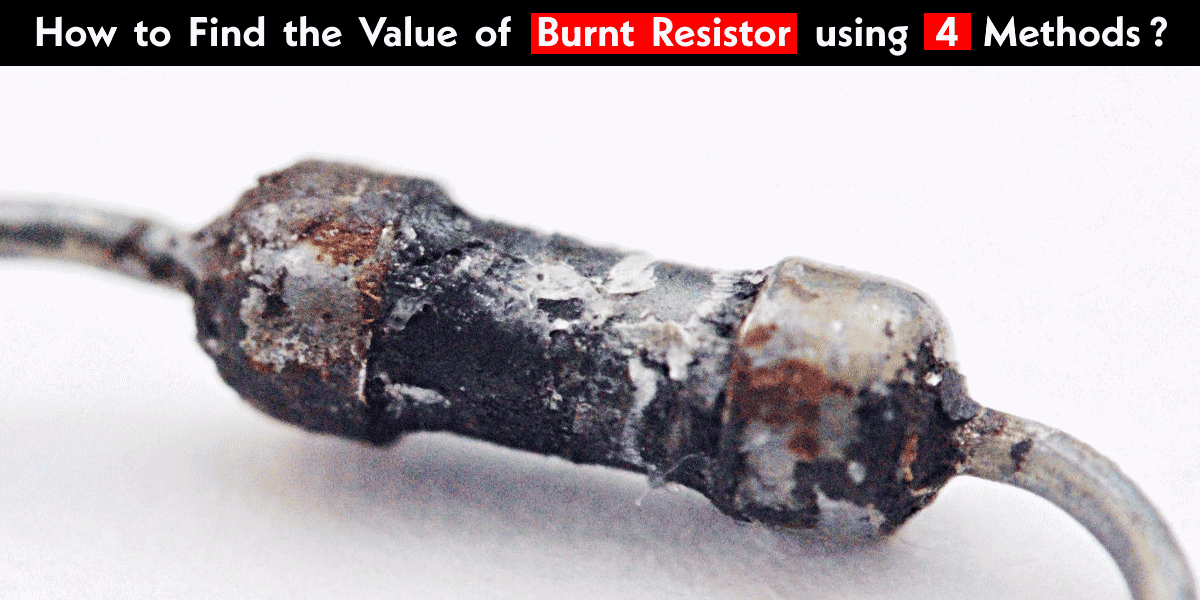# How to Find the Value of Burnt Resistor ( By Four Handy Methods )

## Find the Value of Burnt Resistor by Four Simple Methods

In case of troubleshooting, repairing and designing electric and electronics circuits or damaged circuit boards, we may face this issue where we need to replace the damaged capacitor, diode, resistors etc. In case of resistors, we can find the values of burnt resistors by these four handy methods stated below.Method 1

1. Scarp the outer coating.
2. Clean the burnt section of the resistor.
3. Measure resistance from one end of the resistor to the damaged section.
4. Again measure the resistance from the damaged section to the other end of the resistor.
5. Add these two values of resistances.
6. This is the approximate value of the burnt resistor.
7. Just add a small value of resistance for the damaged section i.e., suppose the value of the burnt resistor was 1k Ω, but you got 970 Ω. So just add 30 Ω, and you will have 1k Ω.

Related Post: How to check a Capacitor by 6 Simple Methods

Method 2

This method can also be used for finding the value of  burnt resistor(s) (also, may be applied on connected resistors in the circuit) in case you don’t know about Resistance Color Coding.

1. Connect resistor to multimeter and measure voltage drop across the burnt resistor.
2. Now measure the current flowing into the resistor.
3. Multiply both values and you will get the wattage of Resistor (As P = VI i.e. Ohm’s law).
4. This wattage must be less than the wattage of the resistor being replaced.Method 3

This method could be used better if you know the expected output voltage of the circuit and you have a set of resistors having the same wattage as the burnt resistor. Perform this method if you don’t know the value of the resistor.

1. Start with a high value of resistance and temporarily connect this resistor instead of burnt resistor.
2. Measure the expected output voltage of the circuit.  If you obtained the same voltage as expected voltage then you have done.
3. If you don’t know about the expected voltage, then keep reducing the value of the resistor until you are satisfied with the work of the circuit for which purpose it was designed for.

Related Post: How to Test a Relay? Checking SSR & Coil Relays

Method 4

Another method which is not always the case is that the value of resistors are already printed on the PCB. In case of a burnt resistor, just look at the printed circuit board (PCB) and look for the value of the resistor printed on it. If not, you may follow the above methods (1-3) instead.Related Post: How to Test a Diode using Digital & Analog Multimeter  4 Ways.

Let us know in the comment below if you know an additional method to find the value of burnt resistors.

Related Posts

### Electrical Technology

1. NISHA BARAIKAR says:

Thats the easy way to explain realy good

2. nestor villanueva says:

nice explanation.done well

3. gidieon says:

A non centre tap transformer outputs 23v dc.

How do I find the amp output.

Thanks

5. Vali Kabini says:

How do i find the resistance value of a burnt redistor?

6. Stanley Aristotle says:

My odds has been interestingly relieved thro’ this…”tnapeq”

7. manoj anand says:

It’s very good and we can use in various places.such as VID.

8. Johnson says:

I have a new idea, good resistor series connect with the burnt resistor and then mesure the resistor value notify then mines(-) the good resistance value from notified value due to the remaining value is that

1. Electrical Technology says:

:P